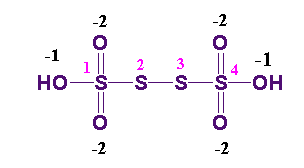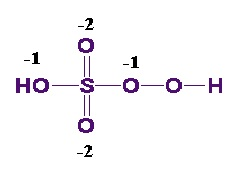# Oxidation State

## What is Oxidation State?

The oxidation state of an atom (sometimes referred to as the oxidation number) in a chemical compound provides insight into the number of electrons lost it and, therefore, describes the extent of oxidation of the atom. The oxidation state of an atom can be defined as the hypothetical charge that would be held by that atom if all of its bonds to other atoms were completely ionic in nature. For example, the oxidation state of carbon in CO2 would be +4 since the hypothetical charge held by the carbon atom if both of the carbon-oxygen double bonds were completely ionic would be equal to +4 (each oxygen atom would hold a charge of -2 since oxygen is more electronegative than carbon).

Oxidation state and oxidation number are terms frequently used interchangeably. They are the quantities which describe the number of electrons lost in an atom. Ideally, the oxidation state is either zero, positive, or negative.## Discovery and Representation

Antoine Lavoisier was the first to use the term oxidation to denote the reaction between a substance and oxygen. Later, it was noticed that when the substance is oxidized it loses electrons. Therefore, the elucidation diversified to include other reactions as well where the electrons are lost, irrespective of the involvement of oxygen atom.

The value of the oxidation state usually constitutes integers. Sometimes the oxidation state is a fraction. For example,

• The element iron in Fe3O4 (magnetite) is 8/3.

## What is Oxidation?

A chemical reaction which includes the movement of electrons is called oxidation. A substance which donates electrons is said to be oxidized. For example,

• The reaction between iron and oxygen – When iron (Fe) reacts with oxygen it forms rust because iron loses electrons and oxygen gains electrons.

## Lowest and Highest Oxidation States

The oxidation state of an atom is not regarded as the real charge of the atom. In a chemical reaction if there is an increase in oxidation state then it is known as oxidation whereas if there is a decrease in oxidation state, it is known as reduction. The lowest known oxidation state is −4, for carbon in CH4 (methane). The highest known oxidation state is +9 in the tetroxoiridium (IX).

## How to calculate Oxidation Number?

To calculate oxidation number we need to understand and follow certain rules. There are six rules:

• Each atom in an element either in its free or uncombined state holds up an oxidation number of zero. Clearly, each atom in H2, Cl2, P4, Na, Al, O2, O3, S8, and Mg, has an oxidation number zero.
• The oxidation number of ions which comprise of only one atom is equal to the actual charge on the ion.
• In most of the compounds, the oxidation number of oxygen is –2. There are two exceptions here.
• Peroxides- Every oxygen atom is allocated an oxidation number of –1. Example, Na2O2
• Superoxides- Every oxygen atom is allocated an oxidation number of –(1/2). Example, KO2
• Oxygen is bonded to fluorine- Example, dioxygen difluoride where the oxygen atom is allocated an oxidation number of +1.
• Hydrogen’s oxidation number is +1, excluding when it is bonded to metals containing two elements. For example, CaH2, its oxidation number equals to –1.
• Fluorine and other halogens have an oxidation number –1 when they appear as halide ions in their compounds. When iodine, chlorine, and bromine are combined with oxygen, their oxidation number is positive.
• When the oxidation number of the atoms of a compound are added together the algebraic sum must be equal to zero. In case of polyatomic ion, when the oxidation number of the atoms of an ion are added together the algebraic sum must be equal to the charge on the ion. Consider (CO3)2– the algebraic sum of oxidation number of one carbon atom and three oxygen atom is equal to -2.

## Examples of oxidation state Calculation

### (a) Average oxidation number of S in H2S4O6In the structure of H2S4O6 , four S atoms are present the average oxidation state of S atom is

In the above structure

Oxidation state of S1 atom is ⇒ x – 2 – 2 – 1 + 0 = 0 ,  ⇒ x = +5

Oxidation state of S2 atom is ⇒ x = 0

Oxidation state of S3 atom is ⇒ x = 0

Oxidation state of S4 atom is ⇒x – 2 – 2 – 1 + 0 = 0 ,  ⇒ x = +5

The average oxidation state of S is (5+ 0+ 0+ 5)/4 = 10/4 = 2.5

### (b) Oxidation number of S in H2SO5Here the oxidation state of OH = -1

oxidation state of peroxo linkage = -1

oxidation state of oxygen  = -2

Oxidation state of sulphur in H2SO5 ⇒  x – 2 – 2 -1 – 1 = 0  ⇒ x = +6

### (c) Oxidation number of P in H3PO4

In the H3PO4 compound the oxidation number of hydrogen = +1

Oxidation number of oxygen is -2

Oxidation number of P is equal to  ⇒ 3(+1) +x + 4(-2) = 0 ⇒  x  = +5

## Frequently Asked Questions – FAQs

Q1

### Why is it called oxidation?

Antoine Lavoisier used the term “oxidation” to describe the interaction of a material with oxygen. Much later, it was discovered that the material loses electrons when oxidised, and the definition was expanded to encompass any process in which electrons are lost, regardless of whether oxygen is present.

Q2

### Why does Oxygen show oxidation state?

Oxygen is extremely electronegative, and it may quickly absorb electrons to complete its valence shell, giving it an Oxidation state of – 2.

Q3

### What is a positive oxidation state?

If the oxidation number is positive, the atom loses electrons; if the oxidation number is negative, the atom acquires electrons. Calcium has a charge of +2, indicating that it has lost two electrons. The -2 charge of oxygen indicates that it has gained two electrons.

Q4

### Does fluorine show a positive oxidation state?

Fluorine is the periodic table’s most electronegative element. As a result, fluorine always has a negative oxidation state and cannot have a positive one.

Q5

### What is the oxidation state of Cl in CaOCl2?

Chlorine coupled to calcium has an oxidation state of -1. Because oxygen has an oxidation state of -2 and the overall molecule in (OCl) has an oxidation state of -1, the oxidation state of chlorine will be +1.